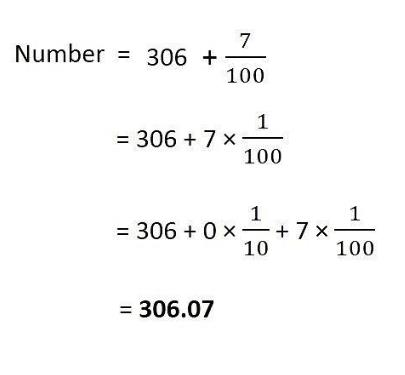Courses

# Test: Decimals

## 10 Questions MCQ Test Mathematics (Maths) Class 6 | Test: Decimals

Description
This mock test of Test: Decimals for Class 6 helps you for every Class 6 entrance exam. This contains 10 Multiple Choice Questions for Class 6 Test: Decimals (mcq) to study with solutions a complete question bank. The solved questions answers in this Test: Decimals quiz give you a good mix of easy questions and tough questions. Class 6 students definitely take this Test: Decimals exercise for a better result in the exam. You can find other Test: Decimals extra questions, long questions & short questions for Class 6 on EduRev as well by searching above.
QUESTION: 1

Solution:
QUESTION: 2

Solution:
QUESTION: 3

### Write 2 rupees and 5 paise as decimal.

Solution:
QUESTION: 4

Decimal form of ‘seven-tenths’ is ________.

Solution:

The decimal 0.7 could be pronounced as SEVEN TENTHS or as ZERO POINT SEVEN.

QUESTION: 5

Decimal notation of one-tenth is ________.

Solution:
QUESTION: 6

0.1 _____ 0.03

Solution:

The correct option is B as 0.1 is greater than 0.03.

QUESTION: 7

Decimal form of ‘Three hundred six and seven-hundredths’ is _______.

Solution:QUESTION: 8

What will be value of (8.5 x 5.8+ 8.5 x 4.2)/(1.7 x 7.6 - 1.7 x 6.6)?

Solution:

(8.5 x 5.8 + 8.5 x 4.2/1.7 x 7.6 - 1.7 x 6.6)

= [8.5 (5.8 + 4.2)/1.7(7.6 - 6.6)]

= (8.5 x 10/1.7 x 1)

= 85/1.7

= 50

QUESTION: 9

Every decimal can be written as a _________.

Solution:
QUESTION: 10

What will be the value of  1 x 0.3 x 0.01 x 0.003?

Solution: